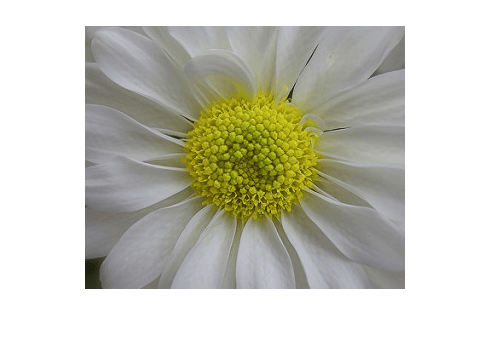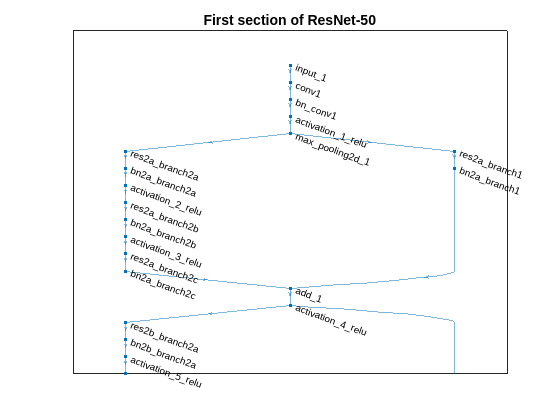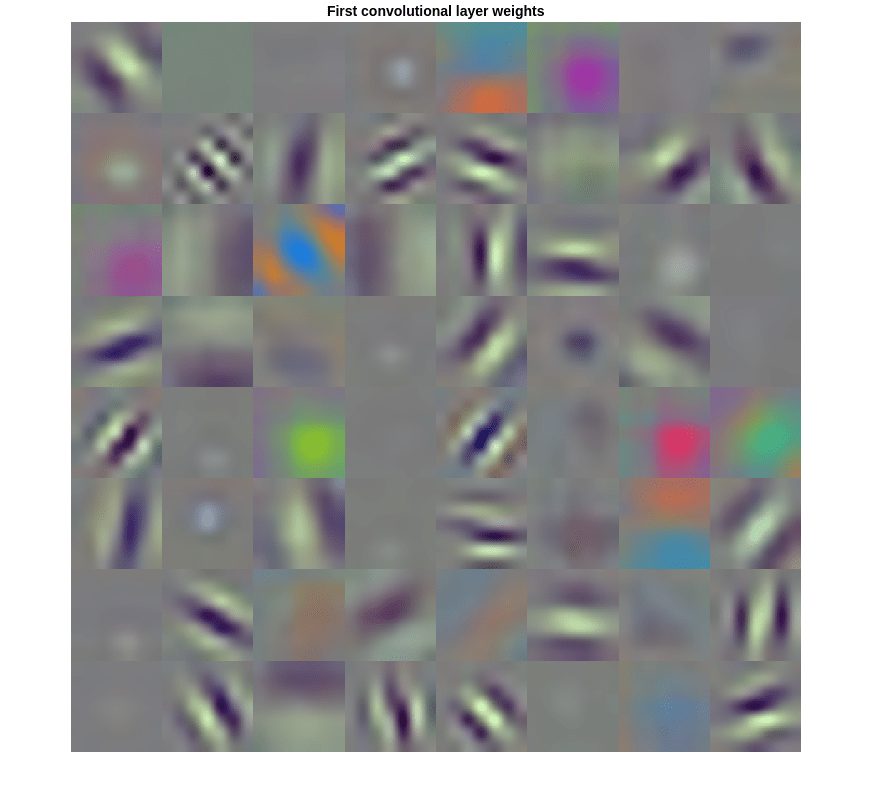# Image Category Classification Using Deep Learning

This example shows how to use a pretrained Convolutional Neural Network (CNN) as a feature extractor for training an image category classifier.

### Overview

A Convolutional Neural Network (CNN) is a powerful machine learning technique from the field of deep learning. CNNs are trained using large collections of diverse images. From these large collections, CNNs can learn rich feature representations for a wide range of images. These feature representations often outperform hand-crafted features such as HOG, LBP, or SURF. An easy way to leverage the power of CNNs, without investing time and effort into training, is to use a pretrained CNN as a feature extractor.

In this example, images from a Flowers Dataset are classified into categories using a multiclass linear SVM trained with CNN features extracted from the images. This approach to image category classification follows the standard practice of training an off-the-shelf classifier using features extracted from images. For example, the Image Category Classification Using Bag of Features example uses SURF features within a bag of features framework to train a multiclass SVM. The difference here is that instead of using image features such as HOG or SURF, features are extracted using a CNN.

Note: This example requires Deep Learning Toolbox™, Statistics and Machine Learning Toolbox™, and Deep Learning Toolbox™ Model for ResNet-50 Network .

Using a CUDA-capable NVIDIA™ GPU with compute capability 3.0 or higher is highly recommended for running this example. Use of a GPU requires the Parallel Computing Toolbox™.

The category classifier will be trained on images from a Flowers Dataset .

```% Location of the compressed data set url = 'http://download.tensorflow.org/example_images/flower_photos.tgz'; % Store the output in a temporary folder downloadFolder = tempdir; filename = fullfile(downloadFolder,'flower_dataset.tgz');```

```% Uncompressed data set imageFolder = fullfile(downloadFolder,'flower_photos'); if ~exist(imageFolder,'dir') % download only once disp('Downloading Flower Dataset (218 MB)...'); websave(filename,url); untar(filename,downloadFolder) end```

Load the dataset using an `ImageDatastore` to help you manage the data. Because `ImageDatastore` operates on image file locations, images are not loaded into memory until read, making it efficient for use with large image collections.

`imds = imageDatastore(imageFolder, 'LabelSource', 'foldernames', 'IncludeSubfolders',true);`

Below, you can see an example image from one of the categories included in the dataset. The displayed image is by Mario.

```% Find the first instance of an image for each category daisy = find(imds.Labels == 'daisy', 1); figure imshow(readimage(imds,daisy))```The `imds` variable now contains the images and the category labels associated with each image. The labels are automatically assigned from the folder names of the image files. Use `countEachLabel` to summarize the number of images per category.

`tbl = countEachLabel(imds)`
```tbl=5×2 table Label Count __________ _____ daisy 633 dandelion 898 roses 641 sunflowers 699 tulips 799 ```

Because `imds` above contains an unequal number of images per category, let's first adjust it, so that the number of images in the training set is balanced.

```% Determine the smallest amount of images in a category minSetCount = min(tbl{:,2}); % Limit the number of images to reduce the time it takes % run this example. maxNumImages = 100; minSetCount = min(maxNumImages,minSetCount); % Use splitEachLabel method to trim the set. imds = splitEachLabel(imds, minSetCount, 'randomize'); % Notice that each set now has exactly the same number of images. countEachLabel(imds)```
```ans=5×2 table Label Count __________ _____ daisy 100 dandelion 100 roses 100 sunflowers 100 tulips 100 ```

There are several pretrained networks that have gained popularity. Most of these have been trained on the ImageNet dataset, which has 1000 object categories and 1.2 million training images. "ResNet-50" is one such model and can be loaded using the `resnet50` function from Neural Network Toolbox™. Using `resnet50` requires that you first install `resnet50`.

```% Load pretrained network net = resnet50();```

Other popular networks trained on ImageNet include AlexNet, GoogLeNet, VGG-16 and VGG-19 , which can be loaded using `alexnet`, `googlenet`, `vgg16`, and `vgg19` from the Deep Learning Toolbox™.

Use `plot` to visualize the network. Because this is a large network, adjust the display window to show just the first section.

```% Visualize the first section of the network. figure plot(net) title('First section of ResNet-50') set(gca,'YLim',[150 170]);```The first layer defines the input dimensions. Each CNN has a different input size requirements. The one used in this example requires image input that is 224-by-224-by-3.

```% Inspect the first layer net.Layers(1)```
```ans = ImageInputLayer with properties: Name: 'input_1' InputSize: [224 224 3] Hyperparameters DataAugmentation: 'none' Normalization: 'zerocenter' NormalizationDimension: 'auto' Mean: [224×224×3 single] ```

The intermediate layers make up the bulk of the CNN. These are a series of convolutional layers, interspersed with rectified linear units (ReLU) and max-pooling layers . Following the these layers are 3 fully-connected layers.

The final layer is the classification layer and its properties depend on the classification task. In this example, the CNN model that was loaded was trained to solve a 1000-way classification problem. Thus the classification layer has 1000 classes from the ImageNet dataset.

```% Inspect the last layer net.Layers(end)```
```ans = ClassificationOutputLayer with properties: Name: 'ClassificationLayer_fc1000' Classes: [1000×1 categorical] OutputSize: 1000 Hyperparameters LossFunction: 'crossentropyex' ```
```% Number of class names for ImageNet classification task numel(net.Layers(end).ClassNames)```
```ans = 1000 ```

Note that the CNN model is not going to be used for the original classification task. It is going to be re-purposed to solve a different classification task on the Flowers Dataset.

### Prepare Training and Test Image Sets

Split the sets into training and validation data. Pick 30% of images from each set for the training data and the remainder, 70%, for the validation data. Randomize the split to avoid biasing the results. The training and test sets will be processed by the CNN model.

`[trainingSet, testSet] = splitEachLabel(imds, 0.3, 'randomize');`

### Pre-process Images For CNN

As mentioned earlier, `net` can only process RGB images that are 224-by-224. To avoid re-saving all the images to this format, use an `augmentedImageDatastore` to resize and convert any grayscale images to RGB on-the-fly. The `augmentedImageDatastore` can be used for additional data augmentation as well when used for network training.

```% Create augmentedImageDatastore from training and test sets to resize % images in imds to the size required by the network. imageSize = net.Layers(1).InputSize; augmentedTrainingSet = augmentedImageDatastore(imageSize, trainingSet, 'ColorPreprocessing', 'gray2rgb'); augmentedTestSet = augmentedImageDatastore(imageSize, testSet, 'ColorPreprocessing', 'gray2rgb');```

### Extract Training Features Using CNN

Each layer of a CNN produces a response, or activation, to an input image. However, there are only a few layers within a CNN that are suitable for image feature extraction. The layers at the beginning of the network capture basic image features, such as edges and blobs. To see this, visualize the network filter weights from the first convolutional layer. This can help build up an intuition as to why the features extracted from CNNs work so well for image recognition tasks. Note that visualizing features from deeper layer weights can be done using `deepDreamImage` from Deep Learning Toolbox™.

```% Get the network weights for the second convolutional layer w1 = net.Layers(2).Weights; % Scale and resize the weights for visualization w1 = mat2gray(w1); w1 = imresize(w1,5); % Display a montage of network weights. There are 96 individual sets of % weights in the first layer. figure montage(w1) title('First convolutional layer weights')```Notice how the first layer of the network has learned filters for capturing blob and edge features. These "primitive" features are then processed by deeper network layers, which combine the early features to form higher level image features. These higher level features are better suited for recognition tasks because they combine all the primitive features into a richer image representation .

You can easily extract features from one of the deeper layers using the `activations` method. Selecting which of the deep layers to choose is a design choice, but typically starting with the layer right before the classification layer is a good place to start. In `net`, this layer is named 'fc1000'. Let's extract training features using that layer.

```featureLayer = 'fc1000'; trainingFeatures = activations(net, augmentedTrainingSet, featureLayer, ... 'MiniBatchSize', 32, 'OutputAs', 'columns');```

Note that the activations function automatically uses a GPU for processing if one is available, otherwise, a CPU is used.

In the code above, the 'MiniBatchSize' is set 32 to ensure that the CNN and image data fit into GPU memory. You may need to lower the 'MiniBatchSize' if your GPU runs out of memory. Also, the activations output is arranged as columns. This helps speed-up the multiclass linear SVM training that follows.

### Train A Multiclass SVM Classifier Using CNN Features

Next, use the CNN image features to train a multiclass SVM classifier. A fast Stochastic Gradient Descent solver is used for training by setting the `fitcecoc` function's 'Learners' parameter to 'Linear'. This helps speed-up the training when working with high-dimensional CNN feature vectors.

```% Get training labels from the trainingSet trainingLabels = trainingSet.Labels; % Train multiclass SVM classifier using a fast linear solver, and set % 'ObservationsIn' to 'columns' to match the arrangement used for training % features. classifier = fitcecoc(trainingFeatures, trainingLabels, ... 'Learners', 'Linear', 'Coding', 'onevsall', 'ObservationsIn', 'columns');```

### Evaluate Classifier

Repeat the procedure used earlier to extract image features from `testSet`. The test features can then be passed to the classifier to measure the accuracy of the trained classifier.

```% Extract test features using the CNN testFeatures = activations(net, augmentedTestSet, featureLayer, ... 'MiniBatchSize', 32, 'OutputAs', 'columns'); % Pass CNN image features to trained classifier predictedLabels = predict(classifier, testFeatures, 'ObservationsIn', 'columns'); % Get the known labels testLabels = testSet.Labels; % Tabulate the results using a confusion matrix. confMat = confusionmat(testLabels, predictedLabels); % Convert confusion matrix into percentage form confMat = bsxfun(@rdivide,confMat,sum(confMat,2))```
```confMat = 5×5 0.8571 0.0286 0.0286 0.0714 0.0143 0.0571 0.8286 0 0.0571 0.0571 0.0143 0 0.7714 0.0714 0.1429 0.0286 0.0571 0.0571 0.8000 0.0571 0 0 0.2000 0.0286 0.7714 ```
```% Display the mean accuracy mean(diag(confMat))```
```ans = 0.8057 ```

### Apply the Trained Classifier On One Test Image

Apply the trained classifier to categorize new images. Read one of the "daisy" test images.

```testImage = readimage(testSet,1); testLabel = testSet.Labels(1)```
```testLabel = categorical daisy ```

Extract image features using the CNN.

```% Create augmentedImageDatastore to automatically resize the image when % image features are extracted using activations. ds = augmentedImageDatastore(imageSize, testImage, 'ColorPreprocessing', 'gray2rgb'); % Extract image features using the CNN imageFeatures = activations(net, ds, featureLayer, 'OutputAs', 'columns');```

Make a prediction using the classifier.

```% Make a prediction using the classifier predictedLabel = predict(classifier, imageFeatures, 'ObservationsIn', 'columns')```
```predictedLabel = categorical daisy ```

### References

 Deng, Jia, et al. "Imagenet: A large-scale hierarchical image database." Computer Vision and Pattern Recognition, 2009. CVPR 2009. IEEE Conference on. IEEE, 2009.

 Krizhevsky, Alex, Ilya Sutskever, and Geoffrey E. Hinton. "Imagenet classification with deep convolutional neural networks." Advances in neural information processing systems. 2012.

 Simonyan, Karen, and Andrew Zisserman. "Very deep convolutional networks for large-scale image recognition." arXiv preprint arXiv:1409.1556 (2014).

 Donahue, Jeff, et al. "Decaf: A deep convolutional activation feature for generic visual recognition." arXiv preprint arXiv:1310.1531 (2013).

 Tensorflow: How to Retrain an Image Classifier for New Categories.